• 浅谈左偏树 左偏树的应用： 左偏树是可并堆的一种实现。它满足堆的性质，并且能在log的时间复杂度下合并两棵左偏树左偏树的定义： 定义1左偏树中的一个节点，如果它的右子树为空，则称它是一个外结点。 ...
参考博客：
浅谈左偏树

左偏树的应用：
左偏树是可并堆的一种实现。它满足堆的性质，并且能在log的时间复杂度下合并两棵左偏树。

左偏树的定义：
定义1 左偏树中的一个节点，如果它的右子树为空，则称它是一个外结点。
定义2 对于左偏树中的一个节点x，到它的子节点中，离它最近的一个外结点经过的边数称为它的距离,记为dist(x)。特别地，外结点的距离为0，空节点(null)的距离为-1。
现在约定一下，本文中出现的左偏树的距离是指，左偏树根节点的距离。
性质1（堆性质） 对于左偏树中的一个非叶节点应满足堆的性质。如果是大根堆，应满足任意非叶节点的左子树和右子树(如果有的话)的根节点的权值大于等于这个节点,即。如果是小根堆则满足应满足任意非叶节点的左子树和右子树(如果有的话)的根节点的权值小于等于这个节点。
性质2（左偏性质） 对于左偏树中的任意节点满足它的左子树的距离大于等于右子树的距离。即。
性质3 左偏树中的任意节点的左子树和右子树(如果有的话)都是左偏树。
由这几条性质可以得出左偏树的定义：左偏树是具有左偏性质的堆有序二叉树。

左偏树性质：
引理1 左偏树中的节点的距离总是满足。
证明 根据定义2有。根据左偏性质可以证得。

现在就来探讨一下当左偏树的距离为  时，左偏树的节点数至少为多少。我们希望节点数尽量少，那应该满足，根据引理1可以得到它们都等于。此时的左偏树就是一棵深度为，所以此时的左偏树的节点个数为。整理得到下面的结论。
定理2 所有距离为k的左偏树中，节点最少的是满二叉树，且节点数为。

当我知道一棵左偏树有n个节点，我能否计算出它的最大深度？答案是肯定的。
推论3 一棵节点数为n的左偏树，它的距离至多为。
证明 根据定理2有。因此  。因为k为整数，所以。因此定理得证。

现在再做一个临时的约定，称从左偏树根节点一直访问右子树，直到不能访问位置所有经过的边和点形成的链为最右链。

引理4 左偏树的最右链恰好有1个外结点。
证明 显然存在一个外结点，不然这一定不是一棵树。假设存在两个，对于深度浅一点的外结点它存在右子树(不然没有后文了)，但是不存在左子树(不然它不是外结点)，与左偏性质矛盾，所以定理得证。

定理5 一棵有n个节点的左偏树的最右链，至多有个节点。
证明 根据推论3有最右链的至多有个非外节点，又根据引理4，可以得知最右链至多有个节点。因此定理得证。

模板：
#include<bits/stdc++.h>
using namespace std;

typedef long long ll;

const int MAX = 1e5 + 10;

//左偏树结构体定义
struct tree {
int l, r;
int fa, dis, val;
}tr[MAX];

int n, m;

//并查集
int finds(int x)
{
return tr[x].fa == -1 ? x : tr[x].fa = finds(tr[x].fa);
}

//合并
//插入操作相当于一个节点的左偏树和另一棵左偏树进行合并
int merge(int x, int y)
{
if (x == 0 || y == 0)	return x + y;  //如果为0的话，就说明是空子树，根节点当然就是另一节点了
if (tr[y].val > tr[x].val)  swap(x, y);  //始终往右子树进行插入
tr[x].r = merge(tr[x].r, y);
tr[tr[x].r].fa = x;
if (tr[tr[x].l].dis < tr[tr[x].r].dis) swap(tr[x].l, tr[x].r);   //是否需要左右子树的对换，这样是为了右子树尽量短
if (tr[x].r == 0)  tr[x].dis = 0;   //距离的重新分配
else tr[x].dis = tr[tr[x].r].dis + 1;
return x;
}

//删除
int del(int root)
{
int l = tr[root].l;
int r = tr[root].r;
tr[root].l = tr[root].r = tr[root].dis = 0;
tr[root].fa = -1;
tr[l].fa = tr[r].fa = -1;  //删除root根节点
return merge(l, r);       //这样一来相当于分裂成了两棵子树，重新进行合并，最后返回值为合并后的根节点
}

//初始化
void init()
{
for (int i = 1; i <= n; i++) {
tr[i].l = tr[i].r = tr[i].dis = 0;
tr[i].fa = -1;
}
}


例题：
hdu 1512 Monkey King

题意：
有n只猴子，每只猴子都有一个能力值，一开始互不认识，当两只不认识的猴子碰到一起，他们就会分别找他们朋友里最强的猴子来和对面决斗，一次决斗后，决斗的两只猴子能力值都除以2，打完一次后，这两派猴子就都是朋友了。现在有m个询问，每次给定两只猴子x，y，如果x，y是一派的，就输出-1，否则就按上述规则进行决斗，并输出决斗完，他们这一派中的能力最大值。

思路：
左偏树模板题。用并查集维护它们是不是一派的，左偏树维护大根堆。如果不是一派的，那么就用x，y所在左偏树的根节点（能力最大值）来互相决斗，决斗完，其能力值/2，先在它们对应的左偏树中删掉它们，再插入（维护左偏树的性质）。最后再进行合并。输出合并后根节点的能力值即可。

Code：
#include<bits/stdc++.h>
using namespace std;

typedef long long ll;

const int MAX = 1e5 + 10;

struct tree {
int l, r;
int fa, dis, val;
}tr[MAX];

int n, m;

int finds(int x)
{
return tr[x].fa == -1 ? x : tr[x].fa = finds(tr[x].fa);
}

int merge(int x, int y)
{
if (x == 0 || y == 0)	return x + y;
if (tr[y].val > tr[x].val)  swap(x, y);
tr[x].r = merge(tr[x].r, y);
tr[tr[x].r].fa = x;
if (tr[tr[x].l].dis < tr[tr[x].r].dis) swap(tr[x].l, tr[x].r);
if (tr[x].r == 0)  tr[x].dis = 0;
else tr[x].dis = tr[tr[x].r].dis + 1;
return x;
}

int del(int root)
{
int l = tr[root].l;
int r = tr[root].r;
tr[root].l = tr[root].r = tr[root].dis = 0;
tr[root].fa = -1;
tr[l].fa = tr[r].fa = -1;
return merge(l, r);
}

int main()
{
while (scanf("%d", &n) != EOF)
{
for (int i = 1; i <= n; i++) {
tr[i].l = tr[i].r = tr[i].dis = 0;
tr[i].fa = -1;
scanf("%d", &tr[i].val);
}
scanf("%d", &m);
while (m--) {
int x, y;
scanf("%d%d", &x, &y);
int fx = finds(x);
int fy = finds(y);
if (fx == fy) {
printf("-1\n");
continue;
}
tr[fx].val /= 2;
//插入操作相当于一个节点的左偏树和另一棵左偏树进行合并
int xx = merge(del(fx), fx);
tr[fy].val /= 2;
int yy = merge(del(fy), fy);
printf("%d\n", tr[merge(xx, yy)].val);
}
}
return 0;
}


展开全文• 左偏树实现c++代码实现，支持查找，删除等多种不同操作，还有建树操作
• 随机生成两棵树的节点，按照插入节点的方法形成两棵左偏树，分别以层次遍历的方法输出这两棵树，然后按照合并算法，再输出合并后的左偏树
• 左偏树入门 数据结构与算法分析 左​偏​树​，重​庆​一​中​，​周​哲​立。
• 左偏树总结
【介绍】  左偏树(Leftist Tree)是一种可并堆的实现。左偏树是一棵二叉树，它的节点除了和二叉树的节点一样具有左右子树指针( left, right)外，还有两个属性，键值和距离(dist)。
【流程】
合并两棵树:  1.有两棵左偏树a,b，如果一个是空树则返回另一个。  2.根据堆的性质判断是否交换插入树和被插入树。  3.用递归将a,b合并。  4.交换后如果不满足左偏性质则交换左右儿子。  5.更新a的距离，并回a。
Ztree* Join(Ztree* a,Ztree* b)
{
if (a==Null) return b; if (b==Null) return a;
if (b->x<a->x||(b->x==a->x&&b->id<a->id)) swap(a,b);// 根据堆的性质判断是否交换插入树和被插入树。
a->son=Join(a->son,b);
if (a->son->dis<a->son->dis) swap(a->son,a->son);//确保满足左偏性质。
if (a->son==Null) a->dis=0; else a->dis=a->son->dis+1;//更新距离。
return a;
}
其实我们可以证明左偏树的复杂度，先假设最坏的情况，树是完全二叉树，左右儿子距离相同，那么复杂度就是log2(n),但是实际上这种情况是不成立的，因为对于每一个点左儿子的距离严格小于右儿子。所以复杂度小于log2(n)。
删除堆顶：
Ztree* Delete(Ztree* a)
{
return Join(a->son,a->son);//合并左右两棵子树相当于删除堆顶。
}
所以左偏树是一个很高效的算法。
【例题】  洛谷3377
展开全文数据结构
• 左偏树 左偏树是可合并的二叉堆，首先满足所有的堆的性质，其外，它还可以合并。 左偏树的树节点需要保存的信息有： 1.左右子树节点编号 2.此节点到有空子结点(子节点数不足2个的节点)的结点的最短距离dist 3...
左偏树
左偏树是可合并的二叉堆，首先满足所有的堆的性质，其外，它还可以合并。
左偏树的树节点需要保存的信息有：
1.左右子树节点编号
2.此节点到有空子结点(子节点数不足2个的节点)的结点的最短距离dist
3.自身权值
性质
左偏树除了堆的所有性质，它还要满足的重要的性质就是“左偏”。
左偏
这个性质保证了它的操作都是O(logn)的。
左偏就是每个节点的左子节点的dist不小于右子节点的dist
（但并不代表左子节点数一定不小于右子节点数），
那么可知dist[i] =dist[rc[i]]+1；
如图（圈内是节点权值，蓝字就是dist值。）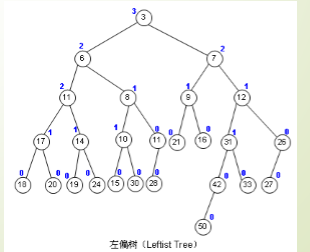操作
堆可以做到的是：插入（O(logn)），查询最值（O(1)），删除堆顶（O(logn)）；  对于左偏树，这些操作都是基于合并的（除了查询最值），而且复杂度都仍然是O(logn)。  左偏树合并操作合并的是两棵左偏树，对于堆的插入，就是合并一棵树和一个节点，对于堆的删除，就是合并根的两棵子树。  合并过程
以小根堆为例，
合并A, B两个堆
如果 A < B（这个不满足的话swap(a,b)）and两棵树的节点没有包含关系(就是没有相同的节点)。
比较B和A的右子树大小，
如果B  <  A的右子树，那么swap B和A的右子树，
接着将B看成刚刚的A，继续swap；
如果B>A的右子树，那么继续找这颗树的右子树。
而这样可能会破坏左偏的性质，
所以需要在回溯的过程中维护左偏性质，
通过交换左右子树完成。
总的来说，左偏树的核心操作，合并(merge)，是在右子树上进行的，
又因为要保证每个节点的左子节点的dist不小于右子节点的dist，
而且有dist[i] =dist[rc[i]]+1，
所以一棵左偏树的效率是O(logn)的
下面附上图会理解得更清晰：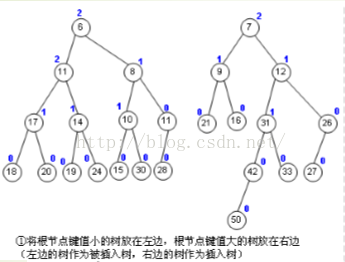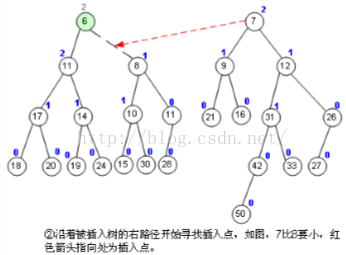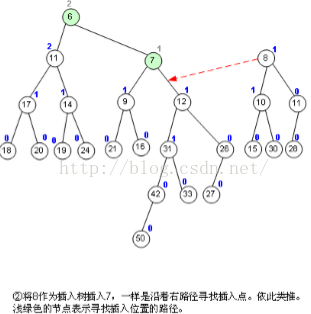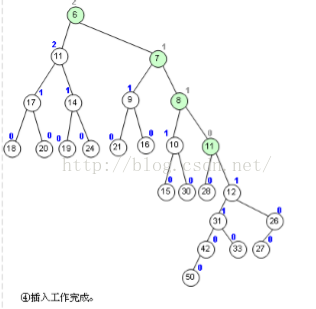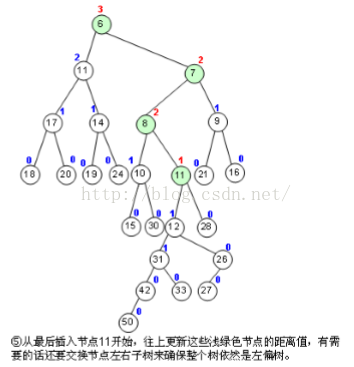// 左偏树模板， 以大根堆为例
struct node{
int w, lc, rc, h;
}t[M];//结构体
//核心操作 ：合并(函数返回值：树根)
int merge(int A, int B){
if(!A||!B) return A+B;
if(t[A].w < t[B].w) swap(A, B);
t[A].rc = merge(t[A].rc, B);
maintain(A); //维护A的一些信息(此模板结构体中并没有)，如：子节点数
if(t[t[A].lc].h < t[t[A].rc].h) swap(t[A].lc, t[A].rc);
if(t[A].rc) t[A].h = t[A].rc+1;
else t[A].h = 0;
return A;
}
//插入
void insert(int A, int x){
cnt++;
t[cnt].w = x;
merge(A, cnt);
}
//删除
void del(int A){
merge(t[A].lc, t[A].rc);
} 
展开全文• 一、左偏树介绍 左偏树(Leftist Tree) 是一种可并堆(Mergeable Heap) ，它除了支持优先队列的三个基本操作（插入，删除，取最小节点），还支持一个很特殊的操作——合并操作。 左偏树是一棵堆有序(Heap Ordered)...
一、左偏树介绍
左偏树(Leftist Tree) 是一种可并堆(Mergeable Heap) ，它除了支持优先队列的三个基本操作（插入，删除，取最小节点），还支持一个很特殊的操作——合并操作。  左偏树是一棵堆有序(Heap Ordered)二叉树。  左偏树满足左偏性质(Leftist Property)。
二、左偏树性质
定义一棵左偏树中的外节点(External Node) 为左子树或右子树为空的节点。  定义节点 i 的距离(dist(i)) 为节点 i 到它的后代中，最近的外节点所经过的边数。  任意节点的左子节点的距离不小于右子节点的距离（左偏性质）。  由左偏性质可知，一个节点的距离等于以该节点为根的子树最右路径的长度。  定理：若一棵左偏树有N个节点，则该左偏树的距离不超过 [log(N+1) ]-1。( [ ] 这里是向下取整…因为打不出来了)。
三、左偏树的操作
3.1、合并
Function Merge(A, B)
If A = NULL Then return B
If B = NULL Then return A
If key(A) > key(B) //保证小根堆的性质
Then swap(A, B)

right(A) ← Merge(right(A), B) //先将A的右子树与B合并
If dist(right(A)) > dist(left(A)) //合并后的右子树距离可能大于左子树距离
Then swap(left(A), right(A)) //交换左右子树

//更新根节点距离
If right(A) = NULL
Then dist(A) ← 0
Else dist(A) ← dist(right(A)) + 1
return A
End Function

合并操作都是一直沿着两棵左偏树的最右路径进行的。  一棵N个节点的左偏树，最右路径上最多有[ log(N+1) ] 个节点。  因此，合并操作的时间复杂度为：O(log N1 + log N2) = O(log N)
3.2、插入新节点
把待插入节点作为一棵单节点左偏树合并两棵左偏树时间复杂度：O(log N)
3.3、删除最小节点
删除根节点合并左右子树时间复杂度：O(log N)
四、左偏树的总结
1、左偏树的特点：（性价比高）
时空效率高编程复杂度低
2、左偏树的应用：（补充二叉堆的不足）
可并堆优先队列
五、模板

\\HYSBZ-2809

#include <iostream>
#include <cstdio>
#include <cstring>
#include <string>
#include <cmath>
#include <algorithm>
#include <vector>

using namespace std;
const int maxn = 100010;

struct heap
{
int l, r, d, w;
} h[maxn];

int n, m, rt[maxn], fa[maxn], sz[maxn];
long long val[maxn], lead[maxn], sum[maxn], ans;
vector<int> G[maxn];

int Merge(int x, int y)
{
if(!x || !y)
return x + y;
if(h[x].w < h[y].w)
swap(x, y);
h[x].r = Merge(h[x].r, y);
rt[h[x].r] = x;
if(h[h[x].l].d < h[h[x].r].d)
swap(h[x].l, h[x].r);
if(h[x].r)
h[x].d = h[h[x].r].d + 1;
else
h[x].d = 0;
return x;
}

int Pop(int x)
{
int l = h[x].l, r = h[x].r;
rt[l] = l;
rt[r] = r;
h[x].l = h[x].r = h[x].d = 0;
return Merge(l, r);
}

int top(int x)
{
return h[x].w;
}

void dfs(int f)
{
for(int i = 0; i < G[f].size(); i++)
{
int u = G[f][i];
dfs(u);
rt[f] = Merge(rt[f], rt[u]);
sum[f] += sum[u];
sz[f] += sz[u];
while(sum[f] > m)
{
sum[f] -= h[rt[f]].w;
sz[f]--;
rt[f] = Pop(rt[f]);
}
}
ans = max(ans, lead[f] * sz[f]);
}

int main()
{
while(~scanf("%d%d", &n, &m))
{
int root;
ans = -1;
for(int i = 1; i <= n; i++)
{
if(fa[i] == 0)
root = i;
G[fa[i]].push_back(i);
h[i].d = h[i].l = h[i].r = 0;
h[i].w = val[i];
rt[i] = i;
sz[i] = 1;
sum[i] = val[i];
}
dfs(root);
printf("%lld\n", ans);
}
return 0;
}

展开全文• 最近学了左偏树，学的时候深感网上没有详细教程之苦，所以自己来写一篇（因为是蒟蒻所以可能写的不是很好） 左偏树是什么？ 左偏，顾名思义，就是往左倾斜，左偏树既满足堆的性质，又满足左偏的性质，实质上，左偏......